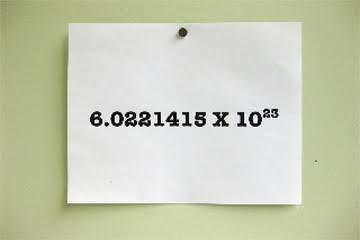Approved & Edited by ProProfs Editorial Team
At ProProfs Quizzes, our dedicated in-house team of experts takes pride in their work. With a sharp eye for detail, they meticulously review each quiz. This ensures that every quiz, taken by over 100 million users, meets our standards of accuracy, clarity, and engagement.
| Written by Hanero
H
Hanero
Community Contributor
Quizzes Created: 89 | Total Attempts: 39,536
Questions: 10 | Attempts: 192SettingsAvogadro's number is the number of particles (atoms or molecules most of the time) that is contained in one mole of a substance. The theory is actually very simple, and you should easily get these questions sorted out, but it depends on whether or not you've used the number before.

• 1.

### What's the name of the man behind the number?

• A.

Stefano

• B.

Amedeo

• C.

Luigi

• D.

Andrea

B. Amedeo
Explanation
The name of the man behind the number is Amedeo.

Rate this question:

• 2.

### What's the symbol of Avogadro's number?

• A.

Q

• B.

L

• C.

T

• D.

Nt

B. L
Explanation
The symbol for Avogadro's number is "L". Avogadro's number, also known as Avogadro's constant, is a fundamental constant in chemistry and physics that represents the number of particles (atoms, molecules, ions, etc.) in one mole of a substance. The symbol "L" is derived from the Italian word "loschimia," which means chemistry. It is used to represent Avogadro's number in various equations and calculations involving the mole concept.

Rate this question:

• 3.

### What's the value of the number?

• A.

6.522140857 × 10^15

• B.

6.022140857 × 10^23

• C.

6.022142357 × 10

• D.

6.111407 × 10^10

B. 6.022140857 × 10^23
Explanation
The given answer, 6.022140857 × 10^23, represents a number in scientific notation. In scientific notation, a number is expressed as a decimal number multiplied by a power of 10. The power of 10 indicates the number of places the decimal point has been moved to the right or left. In this case, the number 6.022140857 is multiplied by 10 raised to the power of 23, which means the decimal point has been moved 23 places to the right. Therefore, the value of the number is a very large number, 6.022140857 followed by 23 zeros.

Rate this question:

• 4.

### Do you know the unit of Avogadro's number?

• A.

Mol

• B.

N

• C.

N^-1

• D.

Mol^-1

C. N^-1
Explanation
The unit of Avogadro's number is N^-1. This is because Avogadro's number represents the number of particles (atoms, molecules, ions, etc.) in one mole of a substance. The unit N^-1 indicates that it is the reciprocal of Avogadro's number, meaning it represents the number of moles per particle. So, N^-1 is the correct unit for Avogadro's number.

Rate this question:

• 5.

### Who first defined Avogadro's number as the number of atoms in one gram per molecule of atomic hydrogen, meaning one gram of hydrogen?

• A.

Niels Bohr

• B.

Jean Baptiste Perrin

• C.

James Clerk Maxwell

• D.

Ernest Rutherford

B. Jean Baptiste Perrin
Explanation
Jean Baptiste Perrin first defined Avogadro's number as the number of atoms in one gram per molecule of atomic hydrogen, meaning one gram of hydrogen.

Rate this question:

• 6.

### What's the number called in German literature?

• A.

Loschmidt Constant

• B.

Japhetic Constant

• C.

Fokker–Planck Constant

• D.

A. Loschmidt Constant
Explanation
The Loschmidt Constant, also known as the Avogadro Constant, is a fundamental constant in physics and chemistry. It represents the number of particles (atoms, molecules, ions) present in one mole of a substance and is often used to convert between the macroscopic and microscopic scales. It is named after the Austrian physicist Johann Loschmidt, who made significant contributions to the understanding of gases and kinetic theory. In German literature, the Loschmidt Constant may be mentioned in the context of scientific discussions or historical references.

Rate this question:

• 7.

### Who actually cemented the theory but named it in the honor or Avogadro?

• A.

Erwin Schrödinger

• B.

Jean Perrin

• C.

Richard Feynman

• D.

Max Planck

B. Jean Perrin
Explanation
Jean Perrin is the correct answer because he was the one who actually cemented the theory, but named it in the honor of Avogadro. Perrin was a French physicist who conducted experiments on Brownian motion, which provided strong evidence for the existence of atoms and molecules. He also made significant contributions to the understanding of Avogadro's law and the determination of Avogadro's number. Therefore, Perrin's work played a crucial role in solidifying the theory and honoring Avogadro's contributions to the field of chemistry.

Rate this question:

• 8.

### When Amedeo Avogadro first propose the theory that dictates the number?

• A.

1834

• B.

1825

• C.

1819

• D.

1811

D. 1811
Explanation
Amedeo Avogadro first proposed the theory that dictates the number in 1811.

Rate this question:

• 9.

### Who first indicated the value of the number?

• A.

Alfred Nobel

• B.

Dmitri Mendeleev

• C.

Linus Pauling

• D.

Johann Josef Loschmidt

D. Johann Josef Loschmidt
Explanation
Johann Josef Loschmidt was the first to indicate the value of the number.

Rate this question:

• 10.

### When did Loschmidt estimate the number's count?

• A.

1912

• B.

1834

• C.

1878

• D.

1865Back to top